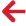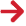﻿ PipeLay > Excel Add-In > Function Reference > Statistics Functions > PLGetStandardDeviation

# PLGetStandardDeviation

Navigation:  PipeLay > Excel Add-In > Function Reference > Statistics Functions >

# PLGetStandardDeviation## PURPOSE

Retrieve the Standard Deviation for the specified parameters in the specified time range from the database.

## HOW IS THIS CALCULATED?

The Standard Deviation is calculated in 2 passes. It is calculated as follows:

Calculate the mean of the values in the range. This is pass 1.

Count the number of items in the specified range, n

For the values in the range, sum the values as sum = sum + ( value - mean)2

Calculate the variance, var = sum / n

The Standard Deviation is calculated as √ var

This function will return a valid result when n > 1.

## SYNTAX

PLGetStandardDeviation( DatabaseName, StartTimeIndex, EndTimeIndex, ParameterType, ItemIndex, DOFNo )

## PARAMETERS

 DatabaseName (Character String) The full path and filename to the relevant database. The file extension is optional. StartTimeIndex (Integer) The start time index, from 1 to the number of solutions in the database. EndTimeIndex (Integer) The end time index, from 1 to the number of solutions in the database. This should be a number higher than StartTimeIndex. ParameterType (Integer) The type of parameter to retrieve data for. See Parameter Types for a complete list. ItemIndex (Integer) The index of the required item. This is parameter dependent e.g. for kinematic parameters, this is a node number. DOFNo (Integer) The DOF or Local node number. This is parameter dependent so the ranges should correspond to what is valid for the parameter.

## OUTPUT

A numerical value containing the standard deviation for the specified parameters or one of the following error codes:

 -999999998 Invalid Database. -999999996 Invalid Parameter Number. -999999995 Invalid Time Index. This may be either the start or end time. -999999990 No Data Available. -999999988 Item Index is out of range. -999999985 Local Node Number or DOF is out of range. -999999980 General Read Error.

Error Codes

Parameters

PLGetEnvelopeData

PLGetMaximumValue

PLGetMeanValue

PLGetMedian

PLGetMinimumValue

PLGetNaiveStandardDeviation

PLGetMode

PLGetRangeValue